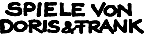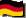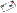Great German Games with a HedgehogOur Games About us Online Games Events Other Contact Order Infos Home  >  Online Games  >  Numfield  >  Another Eval Class# Numfield, Yet another Evaluation Class

This isMark Delano s variation onDon Reble s Evaluation class.
 ```/* This class contains significant modification from Don Reble's Evaluator Class. Although most of the base methods have remained the same (it being quite sound), several key elements have changed. Also, at the end of the EvalX class are array methods that I found myself using over and over again. These should probably be in an entirely separate class, and future updates will probably do just that. As an additional note, many people find my naming schemes confusing. I'm sorry, but I welcome any questions about what is going on. */ public class EvalX { /* ----- data ----- */ // This provides a global storage for the bets, player matches, stake, and totalShares. protected int d_bets[], myMatches, myShares, stake, totalShares; private int d_bonusAndCarry; // The bonus plus carry-over. /* For our purposes, we define "bettor" as an _opponent_ who bet more than zero. That is, a zero-bettor is not a bettor, and I am not a bettor (because I am not my opponent). */ private int d_bettors; // The number of bettors. private int[] d_betHistogram; // BetHistogram[N] has the number of bettors who bet // between 1 and N (inclusive). private int d_totalBet; // The sum of all bettor's bets. private int d_totalShares; // The shares of a bettor is the number of bettors who did not // bet more than he. A bettor must have at least one share, // since he did not bet more than himself. The sum of all // bettors' shares is the Total Shares. /* ----- constructor ----- The only difference from Evaluator is that d_bets is stored globally, to provide for its usage later. */ public EvalX(int bonusAndCarry, int bets[]) { // bets is my bet, which is ignored throughout. // Except in the minimizeBet and maximizeBet methods. d_bets = copyArray(bets); d_bonusAndCarry = bonusAndCarry; /* -- Find the largest bettor's bet, and allocate the histogram. -- */ int maxbet = 0; for (int ix = 1; ix < bets.length; ix += 1) { int bet = bets[ix]; if (bet > maxbet) maxbet = bet; } d_betHistogram = new int[maxbet+1]; /* -- Accumulate the non-zero bets. -- */ // Also, count the bettors, and sum their total bet. // Temporarily, histogram[N] counts the bettors who bet exactly N. d_bettors = 0; for (int ix = 1; ix < bets.length; ix += 1) { int bet = bets[ix]; if (bet > 0) { d_betHistogram[bet] += 1; d_bettors += 1; d_totalBet += bet; } } /* -- Compute the total shares. -- */ // Also, cumulate the histogram. // Compute the total shares, assuming no equal bets. d_totalShares = ((d_bettors + 1) * d_bettors) / 2; // Adjust total shares for equal bets. int sum = 0; for (int bx = 1; bx <= maxbet; bx += 1) { int matches = d_betHistogram[bx]; d_totalShares += ((matches - 1) * matches) / 2; sum += matches; d_betHistogram[bx] = sum; // cumulating the histogram } } public int gain(int mybet) { /* -- Compute the gain I would have when betting Mybet. -- The histogram makes this a constant-time function. Identical to Evaluator, except that initializeGain has been placed in its own method, mostly to provide easier reuse and to store information globally. Obviously not a good idea as far as memory concerns, but the amount taken up is small and only is increased by an int per additional player. */ if (mybet == 0) return 0; initializeGain(mybet); return (stake * myShares) / totalShares - mybet; } public int relativeGain(int ranking[], int mybet){ /* Primary gain method for maximizeBet, and decides ties for bestBet, safestBet and riskiestBet. Determines the gain for each opponent given the player's bet, and weights them using the ranking. This and negativeGain does not return a 0 gain given a 0 bid, as it is conceivable that the 0 bid could bring you a greater weighted gain in these situations. */ initializeGain(mybet); int greatest = findGreatest(ranking); if (greatest == -1) greatest = 0; int gn = ((stake * myShares) / totalShares - mybet)*ranking[greatest]; int myGain = gn; int tempHistogram[] = new int[d_betHistogram.length]; for (int i=1; i 0){ myShares = tempHistogram[d_bets[i]]; myGain += gn - ((stake*myShares/totalShares - d_bets[i])*ranking[i]); } } return myGain; } public int negativeGain(int ranking[], int mybet){ // Fundamentally identical to relativeGain, but it weights in the opposite direction. initializeGain(mybet); int least = findLeast(ranking); if (least == -1) least = 0; int greatest = findGreatest(ranking); if (greatest == -1) greatest = 0; float oppGn = 0; float gn = (float) Math.floor((stake * myShares) / totalShares - mybet)*ranking[greatest]/ranking[least]; oppGn = gn; int tempHistogram[] = new int[d_betHistogram.length]; for (int i=1; i 0){ myShares = tempHistogram[d_bets[i]]; oppGn = gn - (float) Math.floor(stake*myShares/totalShares - d_bets[i])*ranking[greatest]/ranking[i]; } } return (int) Math.ceil(oppGn); } public void initializeGain(int mybet){ // Include my bet and the bonus in the stake. stake = d_totalBet + d_bonusAndCarry + mybet; // Compute my shares, and the number of players I match. myMatches = Math.min(1, mybet); myShares = Math.min(1, mybet); if (mybet >= d_betHistogram.length) { myShares += d_bettors; } else { myShares += d_betHistogram[mybet]; myMatches += d_betHistogram[mybet] - d_betHistogram[Math.max(0, mybet-1)]; // That's why the Histogram has a . } // Compute total shares, including mine. totalShares = d_totalShares + d_bettors + myMatches; } public int bestBet(int ranking[], int maxbet) { /* -- Compute a bet which would have maximized my gain. -- */ // The resolution of tied gains has been changed. // ranking is a means to weight the value of opponents, and // decide ties accordingly. int bestbet = 0; int bestgain = Integer.MIN_VALUE; int relativegain = Integer.MIN_VALUE; for (int bet = 1; bet <= maxbet; bet++) { int ga = gain(bet); int revGa = relativeGain(ranking, bet); if ((ga > bestgain) || (ga == bestgain && revGa > relativegain) ) { bestgain = ga; bestbet = bet; relativegain = revGa; } } return bestbet; } public int maximizeBet(int ranking[], int maxbet){ /* Generally bestBet is the better method to use, but it is possible to use this effectively. It uses the relativeGain for all decision making, instead of just deciding ties. */ int bBet = 0; int bestGain = Integer.MIN_VALUE; for (int i=1; i<=maxbet; i++){ int gn = relativeGain(ranking, i); if (gn > bestGain){ bestGain = gn; bBet = i; } } return bBet; } public int minimizeBet(int ranking[], int maxbet){ /* Basically identical to maximizeBet, except it uses negativeGain, which places the greatest importance on the lowest ranking. A convenience. */ int bBet = 0; int bestGain = Integer.MIN_VALUE; for (int i=1; i<=maxbet; i++){ int gn = negativeGain(ranking, i); if (gn > bestGain){ bestGain = gn; bBet = i; } } return bBet; } /* safestBet and riskiestBet both are similar to bestBet, except that they take two randomly determined similar bet arrays and selects the safest/riskiest course available. Slightly random (although not dangerously so), and much slower and more memory intensive than bestBet. */ public int safestBet(int scores[], int maxbet){ int bBet = 0; int bestGain = Integer.MIN_VALUE; int relativegain = Integer.MIN_VALUE; int lowBets[] = new int[d_bets.length]; int highBets[] = new int[d_bets.length]; for (int i=1; i bestGain || (gn == bestGain && revGn > relativegain)){ bestGain = gn; bBet = i; relativegain = revGn; } } return bBet; } public int riskiestBet(int ranking[], int maxbet){ int bBet = 0; int bestGain = Integer.MIN_VALUE; int relativegain = Integer.MIN_VALUE; int lowBets[] = new int[d_bets.length]; int highBets[] = new int[d_bets.length]; for (int i=1; i bestGain || (gn == bestGain && revGn > relativegain)){ bestGain = gn; bBet = i; relativegain = revGn; } } return bBet; } /* ----------------------------------------------------- */ /* The Following, in no particular order, are various methods dealing with arrays. They are not optimized, but they are generally useful. In some cases it is better to write your own method for a given situation. */ public static int pickProbability(int betArray[]){ /* Randomly selects an array index. Weighted by the size of each value in the array. If all values are 0 it returns -1, an invalid array index. */ int count = sumArray(betArray); if (count == 0) return -1; count = (int) Math.floor(Math.random()*count); for (int i=0; i temp){ temp = inArray[i]; count = i; } return count; } public static int findLeast(int inArray[]){ /* Finds the smallest value in the array, and returns the index. Once again, if all values are 0 it returns -1. */ int temp = Integer.MAX_VALUE; int count = -1; for (int i=0; i

### Example Applications

Well this is Marks MrArrr, which did fairly well and looks so simple based on above tool.
 ```public class MrArrr extends Strategy { public int initial_turn(){ // Picks a number based on participants, and randomizes slightly. return (int) Math.sqrt(opponents) + (int) Math.floor(Math.random()*3-1); } public int turn(int last_turns[], int scores[], int carryOver, int turn_no){ // Creates the Evaluator object, and instantiates it with the previous turn. Evaluator eval = new Evaluator(carryOver + stepBonus, last_turns); // Takes the bestBet from Evaluator and adds 0-2, usually 1 or 2. return eval.bestBet(maxPlayableNumber) + (int) Math.ceil(Math.random()*2); } } ```
(

http://doris-frank.de/numjevlx.html changed 4/16/07, rendered 4/17/07
(c) 1997-2007 by   Doris & Frank    Site  imprint